# Low Speed Doppler Shift

For many low velocity applications such as Doppler velocity measurement, the general form of the Doppler shift is unnecessarily complex. The general Doppler frequency expression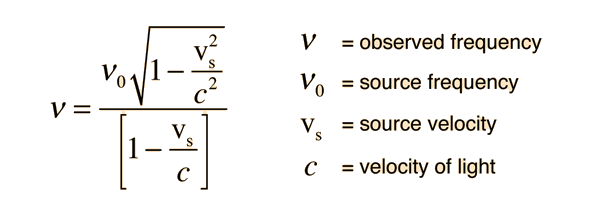can be expanded by the binomial expansion and only the first two terms of the expansion used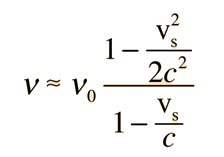Show

When expressed in terms of the frequency shift, this becomes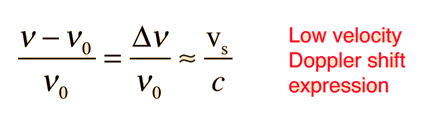Show

 Derivation Calculation
Index

Doppler concepts

 HyperPhysics***** Relativity R Nave
Go Back

# Doppler Expression Expansion

To get a simplified expression for the Doppler frequency expression, the square root in the expressioncan be expanded using the binomial expansion as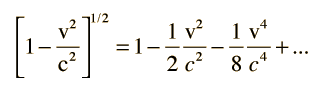For low speeds where v << c, the first two terms give a good approximation of the Doppler shift:Relativistic Doppler effect Calculation
Index

Doppler concepts

 HyperPhysics***** Relativity R Nave
Go Back

# Doppler Calculation

For the relativistic Doppler effect: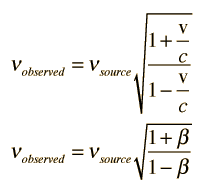v is the relative velocity.
Positive v means approaching.
v = x 108 m/s =c.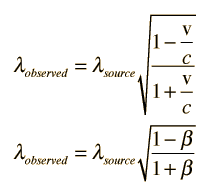For υsource=x10^Hz
υobserved=x10^Hz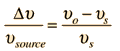=
Δυ=x10^
For λsource=x10^m
λobserved=x10^m
Δλ=x10^m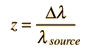==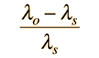For red shift calculations, use negative velocities. Unspecified parameters will default to values for a hydrogen red line source receding at 0.5c, but all values can be changed.

 Relativistic Doppler effect Doppler frequency change Doppler wavelength change
Index

Doppler concepts

 HyperPhysics***** Relativity R Nave
Go Back